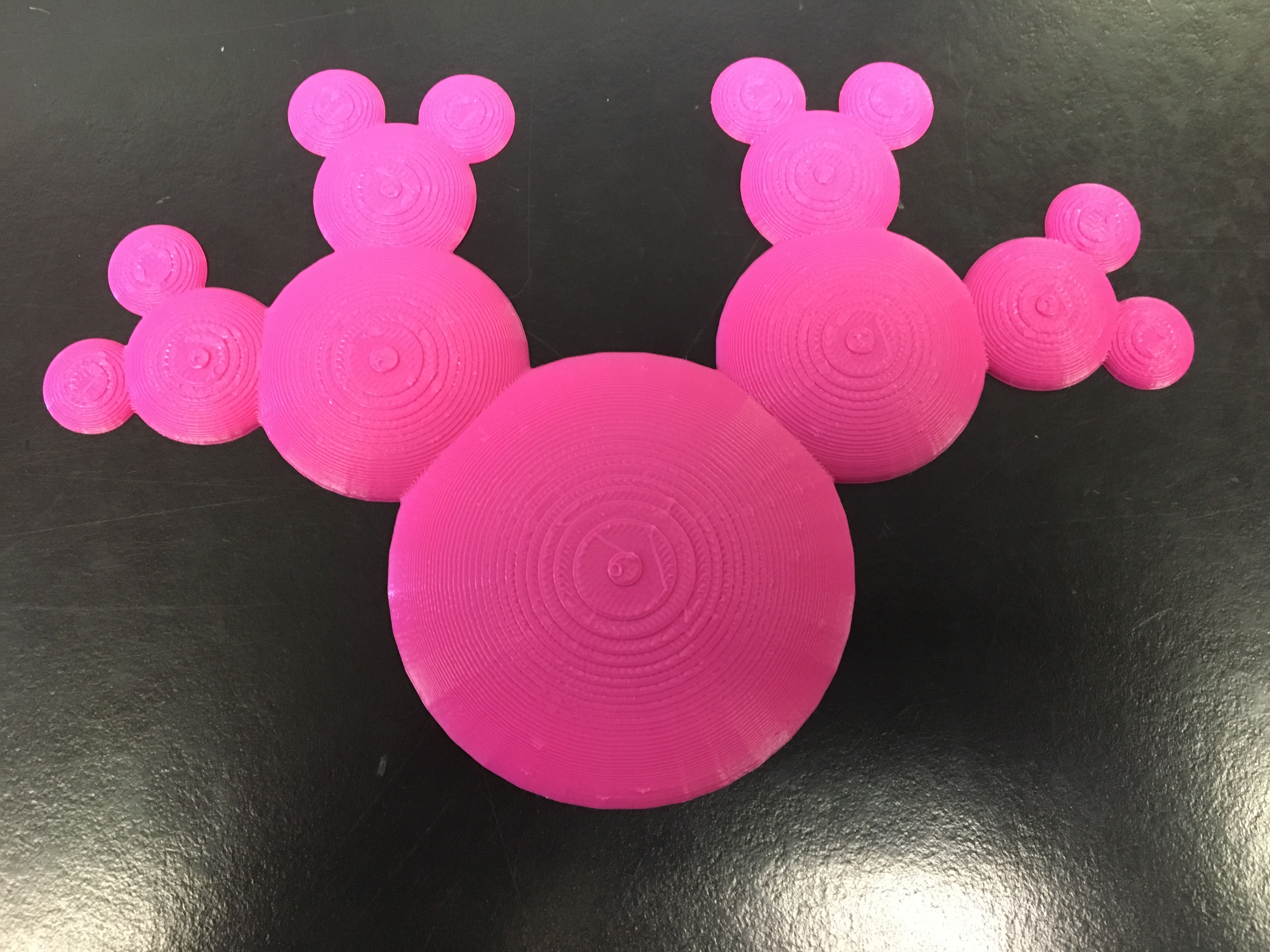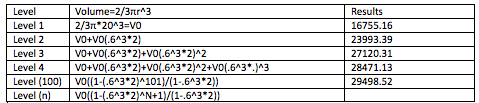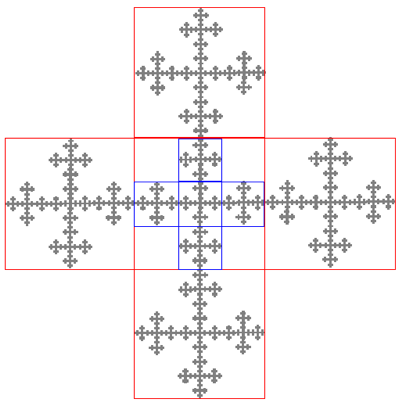# Super Mickey Fractal

### Group Members

Katy Wagner (Writer)

Hunter Simmons (Math Consultant)

Yang Zhou (Designer)

### First Design Iteration

For our first design, our group wanted to do something simple but interesting. At first, we weren’t sure what to create so we got some inspiration by looking up some random and funny things on google. We ended up finding an image that had a bunch of smiley faces which sparked some inspiration for our design. Mathematically, each iteration will add two circles to each prior iteration at a reduced size that is 3/4 smaller than the prior circle. We created this model on Tinkercad with the basic shapes. Although this model was easy to create, it is also very intricate and should be very interesting to create.

We chose this design because it was amusing and looked like it would be fun to make. This design is a fractal because each new iteration continuously decreases by .6 after iteration. The design tool that was mainly used in Tinkercad was the ruler to get exact measurements of the half spheres in the model. We also used the calculator by dividing the initial iteration by 0.6 and then continuously doing the same math for each level.This is only a draft of our model, but hopefully it will be quick to print. We are satisfied with the way it turned out on Tinkercad but the one thing we could change would add two branches at the bottom of the level one circle so there would be four branches instead of two. The model is working the way it was expected to and hopefully the print turns out looking great.

### First Draft Print

This is the Mickey Fractal that we printed on Thursday during class. We had to make the print a little smaller since we did not have enough class time to complete it at its regular size. It took about 40 minutes to print on the Ultimaker. The print turned out looking great but in the future it would be better to print it at its full size.### Volume Calculations

For our design, we calculated the volume of a half of a sphere and each iteration of the design. The formula we used for the volume is 2/3π^3. We use the 2/3 due to the fact that our fractal is only a half sphere rather than a whole sphere.Each level for the calculations includes a decrease in the size of the half sphere by 6/10. But you also double the amount of circles that are being added to each iteration. Our fractal would end up having a finite volume based on our by using a geometric series formula which is later described about. The more levels that are added will ultimately get to a point where the volume levels out and doesn’t increase any more for the levels to come. The formula we used for solving the 100th level as well as N was based on the geometric series detailed below. Luckily we have been able to find out what the maximum volume of our fractal would actually be until hits the finite volume. Formula and Details below.

The formula for Geometric series and more detail within the actual formula can be found at this link “Geometric Series”.

For a simple breakdown of a finite geometric series you can check out Laura Taalman’s Blog!

The Geometric Series formula is a(1/(1-R)). A would be the original size of our level 1 fractal and R= how much you scale down the size of fractal during each iteration. Our geometric series formula would be V0(1/(1-(0.6^3)*2)

Where A=V0 which is equal to the size of our Level 1 which from previous calculations equals 16755.16

16755.16*(1/(1-0.432))

Since R<1 the fractal has a finite area, and after doing computing the above calculations, our fractal has a finite area from which it will reach a maximum of 29498.52…cm^3 in total volume.

### Fractal Dimensions

We were not able to calculate our dimensions due to the larger circle in the middle makes the fractal unequal to split it in a half or thirds. The dimension we are computing are from the Vicsek Fractal. The Vicsek Fractal is scaled down by 1/3 and makes 5 different copies of itself. In calculating the dimensions of the Vicsek Fractal, we first had to find out the size when breaking down the original fractal into smaller counterparts of its self. The Vicsek fractal breaks down into a 3×3 figure shown below in the diagram, which is used in the formula for dimensions of (1/3)^D. The other part of the formula being how many smaller similar parts the original fractal breaks down into, is found by looking at the diagram below, and counting the total smaller vicsek fractals which is a total of 5. This number is used to compute the remaining parts of the formula to get.

(1/3)^d=1/5

3^d=5

D=log_3(5)= (log 5)/(log 3) = 1.46497=Fractal Dimension### Where to Find Our Fractal

Check out our Thingiverse to see our fractal and download the print.

Check out our page on Tinkercad too!

#### 2 Comments on Super Mickey Fractal

1. Can you organize/format the calculation part of your work so that it is easier to read and understand?

2. Can you include a picture of the Vicsek fractal in the dimension section, and refer to it to help readers see what you are calculating?